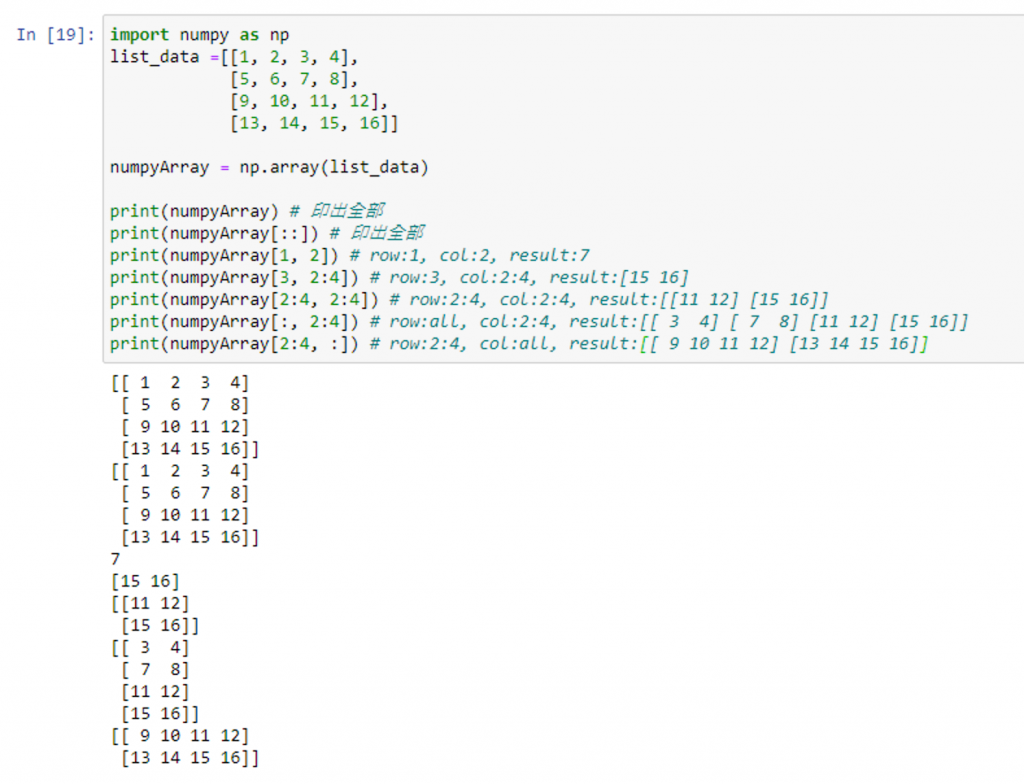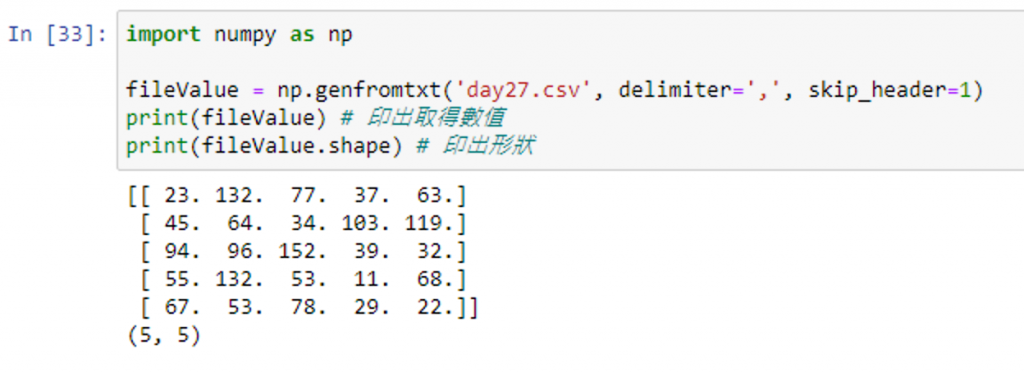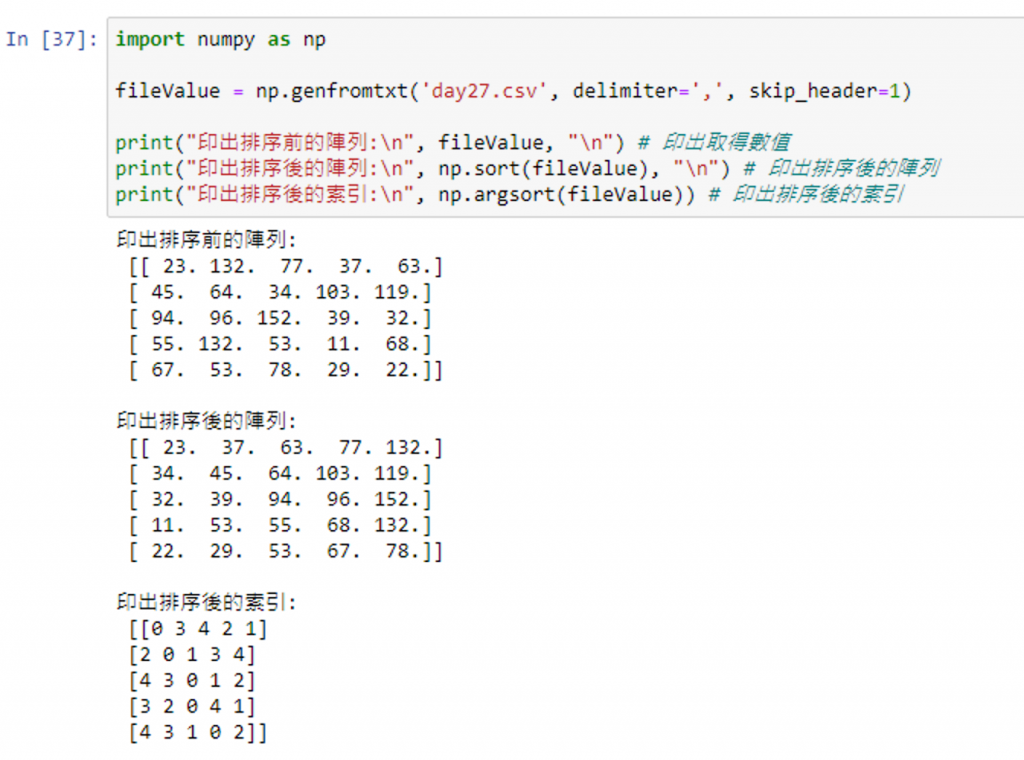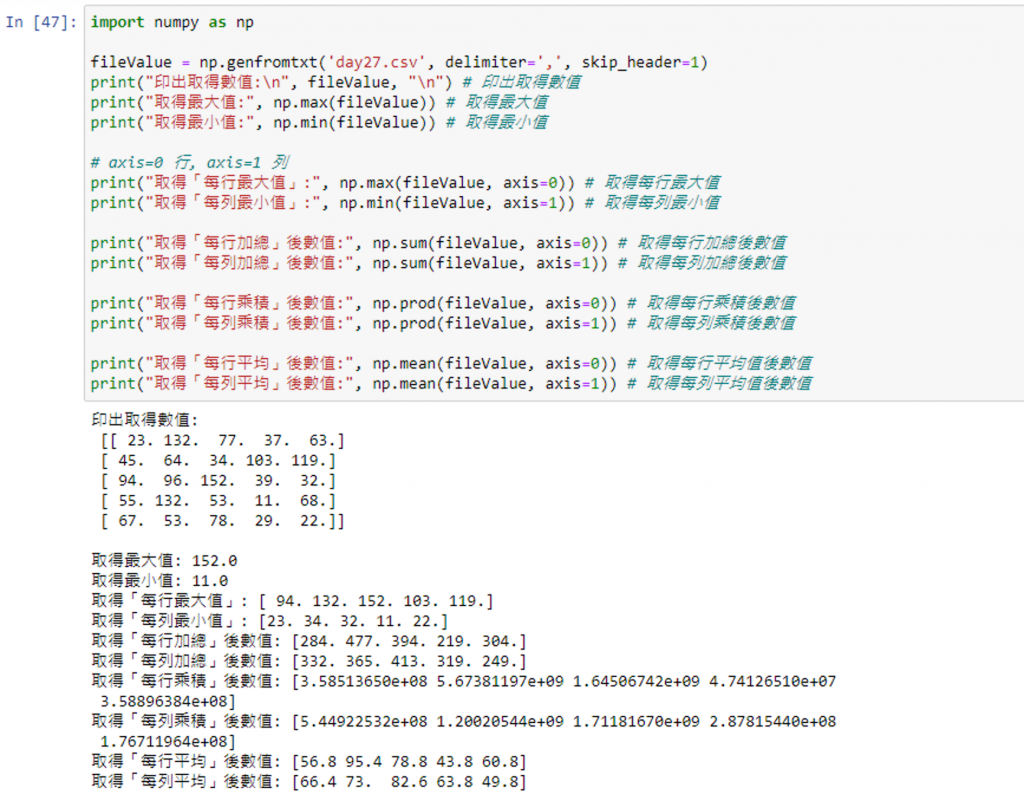#DAY 27
1
Software Development

## [2020鐵人賽Day27]糊裡糊塗Python就上手-Numpy的觀念與運用(下)

### Numpy narray 運用

#### 取得一維陣列的數值

``````narray[索引]
or
narray[起始索引 : 終止索引 [:間隔值]]
``````

``````import numpy as np
arangeValue = np.arange(0,8)

print(arangeValue) # 印出所有值
print(arangeValue[:]) # 印出所有值
print(arangeValue) # 印出索引位置 3 的值
print(arangeValue[3:7]) # 印出索引位置 3~7 的值(不包含7)
print(arangeValue[3:8:2]) # 印出索引位置 3~8 每間隔 2 的值
print(arangeValue[6:2:-1]) # 印出索引 6~2 的值，間隔值為負號代表由右至左讀取
``````#### 取得多維陣列的數值

``````narray[row 索引, col 索引]
``````

(單一索引範圍使用「：」區隔)

``````import numpy as np
list_data =[[1, 2, 3, 4],
[5, 6, 7, 8],
[9, 10, 11, 12],
[13, 14, 15, 16]]

numpyArray = np.array(list_data)

print(numpyArray) # 印出全部
print(numpyArray[::]) # 印出全部
print(numpyArray[1, 2]) # row:1, col:2, result:7
print(numpyArray[3, 2:4]) # row:3, col:2:4, result:[15 16]
print(numpyArray[2:4, 2:4]) # row:2:4, col:2:4, result:[[11 12] [15 16]]
print(numpyArray[:, 2:4]) # row:all, col:2:4, result:[[ 3  4] [ 7  8] [11 12] [15 16]]
print(numpyArray[2:4, :]) # row:2:4, col:all, result:[[ 9 10 11 12] [13 14 15 16]]
````````````narray[matrix 索引, row 索引, col 索引]
``````

``````import numpy as np

import numpy as np
list_data =[
[[1, 2, 3, 4],
[5, 6, 7, 8],
[9, 10, 11, 12],
[13, 14, 15, 16]],
[[14, 18, 13, 12],
[25, 26, 27, 28],
[39, 30, 31, 32],
[43, 44, 45, 46]],
]

numpyArray = np.array(list_data)
print("維度:", numpyArray.ndim)
print("形狀:", numpyArray.shape)
print("數量:", numpyArray.size)
print('\n')
print(numpyArray[::]) # 印出全部
print(numpyArray[1, 3, 2]) # matrix:1, row:3, col:2, result:45
print(numpyArray[0, 1:3, 3]) # matrix:1, row:3, col:2, result:[ 8 12]
print(numpyArray[:, 1:3, 3]) # matrix:all, row:3, col:2, result:[[ 8 12] [28 32]]
``````#### 取得產生的隨機資料(Numpy random)

random 隨機函數 說明
rand() 根據給予的維度形狀，產生 0～1 之間的隨機浮點數資料(不包含 1 )
randn() 根據給予的維度形狀，返回標準常態分佈的隨機浮點數資料
randint(最小值[, 最大值, size]) 返回隨機整數，會依照所設定的最大最小值範圍區間，返回所要求的隨機整數(包含最小值，不包含最大值)
random(size) 根據給予的維度形狀 size，產生 0～1 之間的隨機浮點數資料
random_sample(size) 根據給予的維度形狀 size，產生 0～1 之間的隨機浮點數資料
sample(size) 根據給予的維度形狀 size，產生 0～1 之間的隨機浮點數資料
ranf(size) 根據給予的維度形狀 size，產生 0～1 之間的隨機浮點數資料
choice(array, size[,replace=True]) 從給予的一維陣列中，根據給予的維度形狀 size 返回隨機整數;這邊參數 replace=True 代表會返回重複的資料

``````import numpy as np

print("產生印出 (4x2) 隨機資料:\n", np.random.rand(4,2), "\n")
print("產生印出 (4x2) 常態分布隨機資料:\n", np.random.randn(4,2), "\n")
print("產生印出 3個 0~1 之間的浮點數資料:\n", np.random.random(3), "\n")
print("產生印出 11~25，8 個隨機整數:\n", np.random.randint(11, 25, 8), "\n")
print("產生印出 7個 0~29 不重複的隨機整數:\n", np.random.choice(30, 7, replace=False), "\n")
``````#### 讀取 CSV 取得數值

``````np.genfromtxt('資料檔名', delimiter='分隔符號', skip_header=略過行數)
``````

``````import numpy as np

print(fileValue) # 印出取得數值
print(fileValue.shape) # 印出形狀
``````#### Numpy 排序

numpy.soft()：對陣列中的值進行排序並回傳結果
numpy.argsoft()：對陣列中的值進行排序並回索引

``````import numpy as np

print("印出排序前的陣列:\n", fileValue, "\n") # 印出取得數值
print("印出排序後的陣列:\n", np.sort(fileValue), "\n") # 印出排序後的陣列
print("印出排序後的索引:\n", np.argsort(fileValue)) # 印出排序後的索引
``````#### Numpy 常用運算與統計函數

sum 加總
prod 乘積
mean 平均值
max 最大值
min 最小值

(axis為軸向，axis=0 代表行, axis=1 代表列)

``````import numpy as np

print("印出取得數值:\n", fileValue, "\n") # 印出取得數值
print("取得最大值:", np.max(fileValue)) # 取得最大值
print("取得最小值:", np.min(fileValue)) # 取得最小值

# axis=0 行, axis=1 列
print("取得「每行最大值」:", np.max(fileValue, axis=0)) # 取得每行最大值
print("取得「每列最小值」:", np.min(fileValue, axis=1)) # 取得每列最小值

print("取得「每行加總」後數值:", np.sum(fileValue, axis=0)) # 取得每行加總後數值
print("取得「每列加總」後數值:", np.sum(fileValue, axis=1)) # 取得每列加總後數值

print("取得「每行乘積」後數值:", np.prod(fileValue, axis=0)) # 取得每行乘積後數值
print("取得「每列乘積」後數值:", np.prod(fileValue, axis=1)) # 取得每列乘積後數值

print("取得「每行平均」後數值:", np.mean(fileValue, axis=0)) # 取得每行平均值後數值
print("取得「每列平均」後數值:", np.mean(fileValue, axis=1)) # 取得每列平均值後數值
``````std 標準差
var 變異數
median 中位數
percentile 百分比
ptp 最大值與最小值差值

``````import numpy as np

numpyArray = np.random.randint(50, size=20)

print("隨機產出的數值為:\n", numpyArray)

print("取得「標準差」:", np.std(numpyArray)) # 取得標準差
print("取得「變異數」:", np.var(numpyArray)) # 取得變異數
print("取得「中位數」:", np.median(numpyArray)) # 取得中位數
print("取得「百分比」:", np.percentile(numpyArray, 100)) # 取得百分比
print("取得「最大值與最小值差值」:", np.ptp(numpyArray)) # 取得最大值與最小值差值
``````